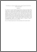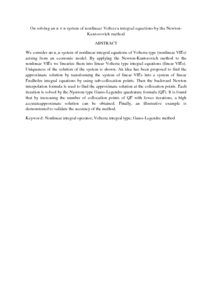# On solving an nxn system of nonlinear Volterra integral equations by the Newton-Kantorovich method

## Citation

Hameed, Hameed Husam and Eshkuvatov, Zainidin K. and Nik Long, Nik Mohd Asri (2016) On solving an nxn system of nonlinear Volterra integral equations by the Newton-Kantorovich method. Science Asia, 42S (1). pp. 11-18. ISSN 1513-1874

## Abstract

We consider an nxn system of nonlinear integral equations of Volterra type (nonlinear VIEs) arising from an economic model. By applying the Newton-Kantorovich method to the nonlinear VIEs we linearize them into linear Volterra type integral equations (linear VIEs). Uniqueness of the solution of the system is shown. An idea has been proposed to find the approximate solution by transforming the system of linear VIEs into a system of linear Fredholm integral equations by using sub-collocation points. Then the backward Newton interpolation formula is used to find the approximate solution at the collocation points. Each iteration is solved by the Nystrom type Gauss-Legendre quadrature formula (QF). It is found that by increasing the number of collocation points of QF with fewer iterations, a high accurate approximate solution can be obtained. Finally, an illustrative example is demonstrated to validate the accuracy of the method.Preview
PDF
On solving an n × n system of nonlinear Volterra integral equations by the Newton-Kantorovich method.pdfView Item